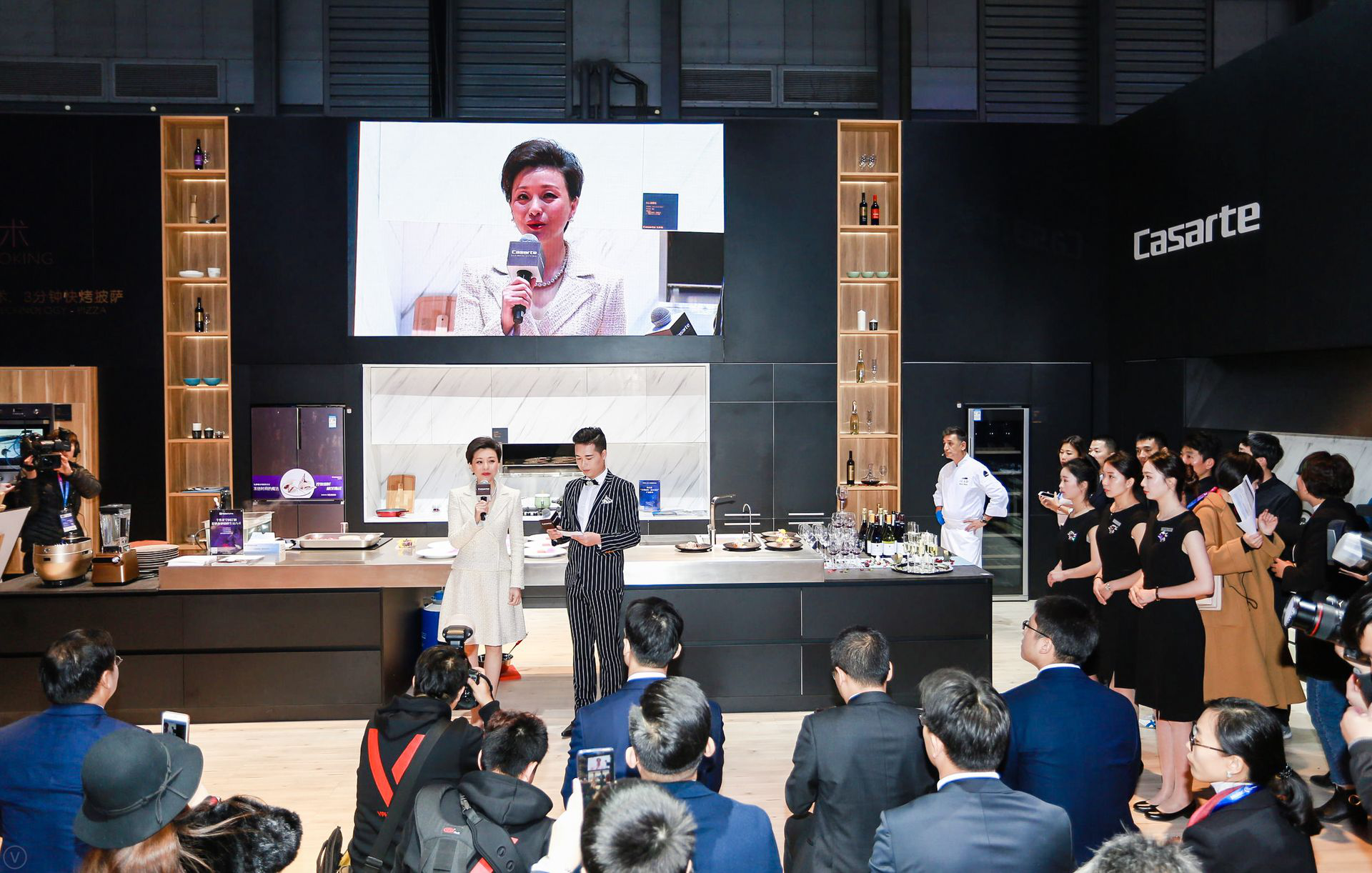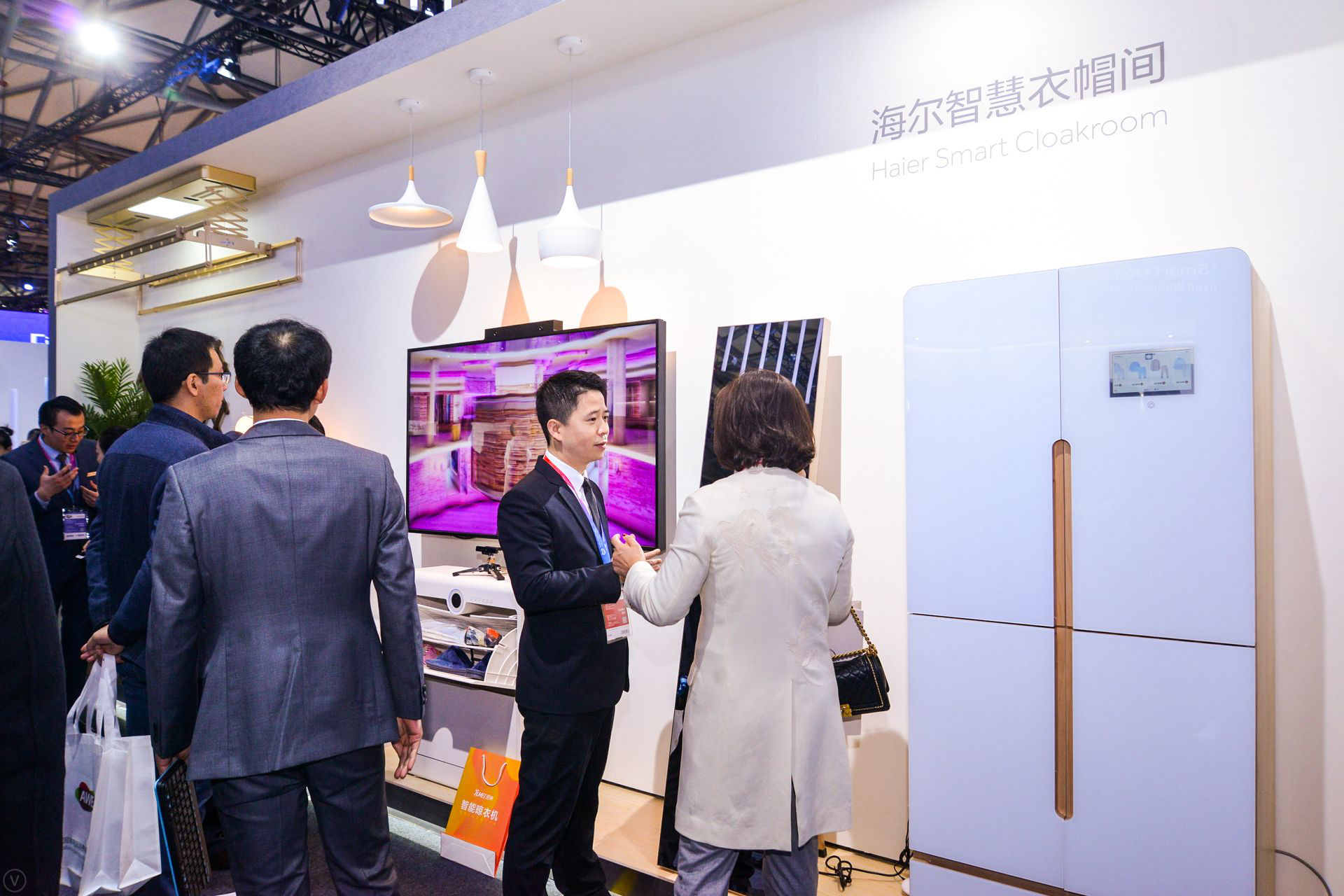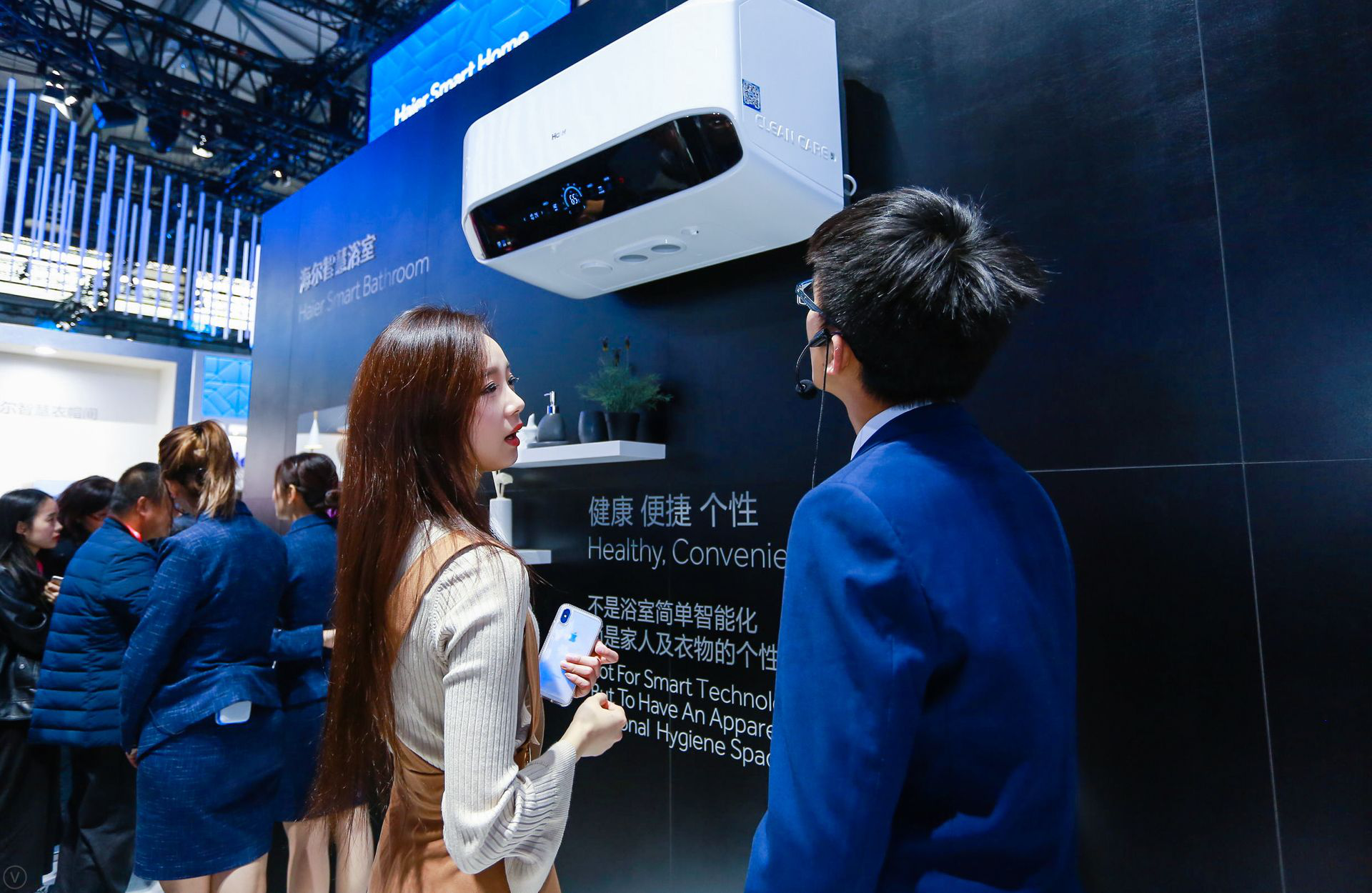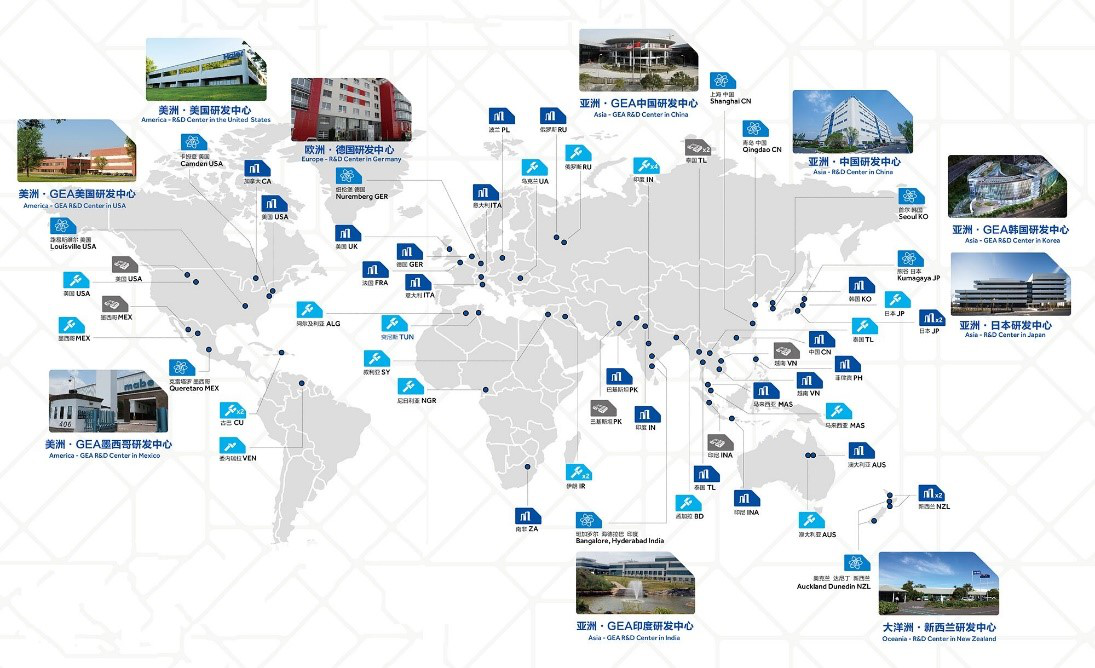|

# 杨澜探秘海尔智慧家庭为品质节打call

东方卫视专题报道：杨澜探秘海尔智慧家庭为品质节打call

3月8日，2018年中国家电及消费电子博览会(AWE)在上海如期举行。海尔在AWE前一天首次发布和展示了目前世界范围内第一个全场景定制化智慧成套方案。与此同时，海尔在全国正式启动以“海尔智慧家庭，美好生活一站购”为主题的品质节活动，旨在让全国用户能享受到智慧科技带来的美好生活。

3月9日，AWE海尔馆还吸引了上海东方卫视和著名主持人杨澜的目光，现场杨澜和广大观众一起探秘海尔全场景定制化智慧成套方案，并进行了长达40分钟的专题直播报道。海尔线下30000家门店、9万块云屏同步直播，10多万海尔员工、客户及媒体和亿万中国用户同时在线观看，向更多中国家庭普及了智慧家庭的美好生活。那么，是什么原因让杨澜和东方卫视在众多家电企业中独独选择海尔呢？

世界最好的智慧家庭解决方案为美好生活按下“启动键”

当前，随着80后、90后逐渐成为社会消费主体，加之互联网技术的快速崛起，居民的消费方式与消费需求开始发生转变，科技化、智能化等多元化需求特点也渐趋显著，智能家居的概念开始深入到日常生活工作中。因此，在今年的品质节上，海尔带来了世界上最好的智慧家庭解决方案，让人们提前享受美好生活。

你是否想过这样的生活场景：下班回到家，已经有人帮你清扫好了房屋，净化好了空气，并在你开门的瞬间缓缓拉上窗帘，打开空调，把室温调整到最合适的温度；步入厨房，有一个机器人助手已经在待命，随时帮你指挥烤箱、灶具做餐；浴室内，热水器已经准备好了热水，等待你洗浴……相比下班之后被孩子折腾的一团乱的家，这样的生活应该是不少人的向往。

在2018AWE上，海尔发布了行业首个“4+7+N”全场景定制化智慧成套方案，基于智慧客厅、智慧厨房等4大持续迭代的物理空间，带来了空气、用水、洗护等7大全屋解决方案，破解了行业难互通、不主动、未成套、服务碎片化的4大难题，让智慧生活进入家庭不再困难。通过在全国同步开展的品质节活动，海尔将这样的智慧生活场景由展会复制到卖场，消费者可以通过线上顺逛平台以及线下智慧家庭体验店体验购买。3个一站式能力为智慧家庭“飞入寻常百姓家”装上加速引擎

提起购买家电，许多消费者可能遇到过这样的问题：家里往往是东奔西跑买到各种零散的单品才能拼凑起一个智慧家庭，遇到产品问题还需要挨个联系商家解决，让用户费时、费力又费心。因此，在品质节上，作为美好生活提供商的海尔，以一站式成套设计、一站式成套销售、一站式成套服务3个一站式能力，让消费者享受“美好生活一站购”。

一站式成套设计方面，依托海尔迭代升级的3D云设计平台，可提供全屋一键配置的省心设计，以及空气、用水、洗护等7大全屋解决方案的原创颠覆性爆品。比如，在选购家电时，只要说出你所在的小区，海尔就能找到你所在小区的所有户型，5分钟就能推荐一个最适合日常智慧生活场景的解决方案，用户可以在此基础上定制、迭代、升级。

一站式成套销售方面，海尔从今年春季开始，全国三万名导购人员经专业培训，已从卖单品家电的导购转变为卖解决方案的家电顾问。此次品质节还特别推出智慧套、品质套等不同套系，消费者可根据需要选择套系或自由定制，保证全屋智慧方案一次买齐。

一站式成套服务方面，海尔在行业内率先实现一站式端到端的成套定制服务，全流程生命周期管护，1个服务团队1次性解决用户成套家电的任何服务需求，破解了用户多次选择多方下单、多次安装的费心、费钱、费时等痛点，为智慧家庭飞入寻常百姓家扫除障碍。

在3个一站式能力带来的智慧家庭里，你可以享受到这样的智慧生活：当你下班回家，进入厨房准备晚餐时，冰箱上的智慧大屏就会自动开启，根据健康饮食记录主动提醒你今晚应该吃什么，并能根据历史口味需求和时令推荐健康菜谱，还能指挥吸油烟机、烤箱、洗碗机进入工作状态。离开冰箱，走到海尔云厨吸油烟机面前，用户可以根据油烟机上的大屏学习名师菜谱，也能在线购买食材和餐具。烹饪完毕后，一键上传美图分享自己的烘焙乐趣。

在布局一站式成套能力之外，海尔还连通了体验购买渠道，加速智慧家庭落地入户。目前，海尔已经拥有30000家门店，今年还将继续布局6000+智慧家庭体验店。为了进一步升级人们的体验，海尔还将在全国建立10家智慧家庭城市体验中心，这是继去年11月在重庆落地全国首个“智慧家庭城市体验中心”之后的又一次深入布局，能让消费者体验到集海尔旗下所有智能家电联动而成的空气、用水、洗护等智慧生活解决方案，现实呈现全智能、全交互的智慧家庭。同时，线上顺逛平台及其他电商平台也将打造智慧家庭体验中心，“线上+线下”多种渠道方便用户体验购买。探秘“美好生活一站购”背后：海尔不可复制的NO.1实力

海尔以“美好生活一站购”为核心开启了本届品质节活动，而这套全流程整合体系背后，则隐藏着海尔不可复制的全球品牌、科技、制造实力，以及3大平台的协同运作支撑。

中国家电全球化第一品牌的实力不可复制。海尔自出海伊始就选择了一条自主创牌路，形成了海尔、卡萨帝、美国GE Appliances、新西兰 Fisher&Paykel、日本AQUA、统帅6大品牌，以中国家电全球化第一品牌的实力赢获了160多个国家和地区的10亿用户，为智慧家庭的全球落地，积累了非常广阔的“人脉”资源。

原创科技世界第一的实力不可复制。从研发布局来看，海尔依托线上平台HOPE以及线下在全球的10大研发中心，连接全球320万在线资源，为海尔智慧家庭的落地提供不可复制的创新力，而同时，海尔的原创科技被世界模仿了112次。此外，“人单合一”模式下独有的3大平台，则为智慧家庭提供了生态协同支撑。基于顺逛物联网第一社群交互平台，80万微店主构成的“用户传感器”可以零距离交互用户需求；而COSMOPlat工业互联网第一平台，则让智慧家庭产品、服务及N个定制化方案快速、精准落地；依托智慧家庭行业人工智能第一平台U+，海尔可以聚集更多的开发者，实现场景的快速迭代。

在AWE上，海尔向全球展示了其在智慧家庭领域取得的一系列成果，而3月8日至4月8日的品质节活动则加速了将这些颠覆性原创成果落地入户的进程。随着海尔品质节的深入开展，将有越来越多的中国家庭装上“智慧”引擎，越来越多的中国用户享受到“未来的美好生活”。

`声明：本文由入驻焦点开放平台的作者撰写，除焦点官方账号外，观点仅代表作者本人，不代表焦点立场错误信息举报电话： 400-099-0099，邮箱：jubao@vip.sohu.com，或点此进行意见反馈，或点此进行举报投诉。`A B C D E F G H J K L M N P Q R S T W X Y Z
A - B - C - D - E
• A
• 鞍山
• 安庆
• 安阳
• 安顺
• 安康
• 澳门
• B
• 北京
• 保定
• 包头
• 巴彦淖尔
• 本溪
• 蚌埠
• 亳州
• 滨州
• 北海
• 百色
• 巴中
• 毕节
• 保山
• 宝鸡
• 白银
• 巴州
• C
• 承德
• 沧州
• 长治
• 赤峰
• 朝阳
• 长春
• 常州
• 滁州
• 池州
• 长沙
• 常德
• 郴州
• 潮州
• 崇左
• 重庆
• 成都
• 楚雄
• 昌都
• 慈溪
• 常熟
• D
• 大同
• 大连
• 丹东
• 大庆
• 东营
• 德州
• 东莞
• 德阳
• 达州
• 大理
• 德宏
• 定西
• 儋州
• 东平
• E
• 鄂尔多斯
• 鄂州
• 恩施
F - G - H - I - J
• F
• 抚顺
• 阜新
• 阜阳
• 福州
• 抚州
• 佛山
• 防城港
• G
• 赣州
• 广州
• 桂林
• 贵港
• 广元
• 广安
• 贵阳
• 固原
• H
• 邯郸
• 衡水
• 呼和浩特
• 呼伦贝尔
• 葫芦岛
• 哈尔滨
• 黑河
• 淮安
• 杭州
• 湖州
• 合肥
• 淮南
• 淮北
• 黄山
• 菏泽
• 鹤壁
• 黄石
• 黄冈
• 衡阳
• 怀化
• 惠州
• 河源
• 贺州
• 河池
• 海口
• 红河
• 汉中
• 海东
• I
• J
• 晋中
• 锦州
• 吉林
• 鸡西
• 佳木斯
• 嘉兴
• 金华
• 景德镇
• 九江
• 吉安
• 济南
• 济宁
• 焦作
• 荆门
• 荆州
• 江门
• 揭阳
• 金昌
• 酒泉
• 嘉峪关
K - L - M - N - P
• K
• 开封
• 昆明
• 昆山
• L
• 廊坊
• 临汾
• 辽阳
• 连云港
• 丽水
• 六安
• 龙岩
• 莱芜
• 临沂
• 聊城
• 洛阳
• 漯河
• 娄底
• 柳州
• 来宾
• 泸州
• 乐山
• 六盘水
• 丽江
• 临沧
• 拉萨
• 林芝
• 兰州
• 陇南
• M
• 牡丹江
• 马鞍山
• 茂名
• 梅州
• 绵阳
• 眉山
• N
• 南京
• 南通
• 宁波
• 南平
• 宁德
• 南昌
• 南阳
• 南宁
• 内江
• 南充
• P
• 盘锦
• 莆田
• 平顶山
• 濮阳
• 攀枝花
• 普洱
• 平凉
Q - R - S - T - W
• Q
• 秦皇岛
• 齐齐哈尔
• 衢州
• 泉州
• 青岛
• 清远
• 钦州
• 黔南
• 曲靖
• 庆阳
• R
• 日照
• 日喀则
• S
• 石家庄
• 沈阳
• 双鸭山
• 绥化
• 上海
• 苏州
• 宿迁
• 绍兴
• 宿州
• 三明
• 上饶
• 三门峡
• 商丘
• 十堰
• 随州
• 邵阳
• 韶关
• 深圳
• 汕头
• 汕尾
• 三亚
• 三沙
• 遂宁
• 山南
• 商洛
• 石嘴山
• T
• 天津
• 唐山
• 太原
• 通辽
• 铁岭
• 泰州
• 台州
• 铜陵
• 泰安
• 铜仁
• 铜川
• 天水
• 天门
• W
• 乌海
• 乌兰察布
• 无锡
• 温州
• 芜湖
• 潍坊
• 威海
• 武汉
• 梧州
• 渭南
• 武威
• 吴忠
• 乌鲁木齐
X - Y - Z
• X
• 邢台
• 徐州
• 宣城
• 厦门
• 新乡
• 许昌
• 信阳
• 襄阳
• 孝感
• 咸宁
• 湘潭
• 湘西
• 西双版纳
• 西安
• 咸阳
• 西宁
• 仙桃
• 西昌
• Y
• 运城
• 营口
• 盐城
• 扬州
• 鹰潭
• 宜春
• 烟台
• 宜昌
• 岳阳
• 益阳
• 永州
• 阳江
• 云浮
• 玉林
• 宜宾
• 雅安
• 玉溪
• 延安
• 榆林
• 银川
• Z
• 张家口
• 镇江
• 舟山
• 漳州
• 淄博
• 枣庄
• 郑州
• 周口
• 驻马店
• 株洲
• 张家界
• 珠海
• 湛江
• 肇庆
• 中山
• 自贡
• 资阳
• 遵义
• 昭通
• 张掖
• 中卫

1室1厅1厨1卫1阳台

1
2
3
4
5

0
1
2

1

1

0
1
2
3报名成功，资料已提交审核A B C D E F G H J K L M N P Q R S T W X Y Z
A - B - C - D - E
• A
• 鞍山
• 安庆
• 安阳
• 安顺
• 安康
• 澳门
• B
• 北京
• 保定
• 包头
• 巴彦淖尔
• 本溪
• 蚌埠
• 亳州
• 滨州
• 北海
• 百色
• 巴中
• 毕节
• 保山
• 宝鸡
• 白银
• 巴州
• C
• 承德
• 沧州
• 长治
• 赤峰
• 朝阳
• 长春
• 常州
• 滁州
• 池州
• 长沙
• 常德
• 郴州
• 潮州
• 崇左
• 重庆
• 成都
• 楚雄
• 昌都
• 慈溪
• 常熟
• D
• 大同
• 大连
• 丹东
• 大庆
• 东营
• 德州
• 东莞
• 德阳
• 达州
• 大理
• 德宏
• 定西
• 儋州
• 东平
• E
• 鄂尔多斯
• 鄂州
• 恩施
F - G - H - I - J
• F
• 抚顺
• 阜新
• 阜阳
• 福州
• 抚州
• 佛山
• 防城港
• G
• 赣州
• 广州
• 桂林
• 贵港
• 广元
• 广安
• 贵阳
• 固原
• H
• 邯郸
• 衡水
• 呼和浩特
• 呼伦贝尔
• 葫芦岛
• 哈尔滨
• 黑河
• 淮安
• 杭州
• 湖州
• 合肥
• 淮南
• 淮北
• 黄山
• 菏泽
• 鹤壁
• 黄石
• 黄冈
• 衡阳
• 怀化
• 惠州
• 河源
• 贺州
• 河池
• 海口
• 红河
• 汉中
• 海东
• I
• J
• 晋中
• 锦州
• 吉林
• 鸡西
• 佳木斯
• 嘉兴
• 金华
• 景德镇
• 九江
• 吉安
• 济南
• 济宁
• 焦作
• 荆门
• 荆州
• 江门
• 揭阳
• 金昌
• 酒泉
• 嘉峪关
K - L - M - N - P
• K
• 开封
• 昆明
• 昆山
• L
• 廊坊
• 临汾
• 辽阳
• 连云港
• 丽水
• 六安
• 龙岩
• 莱芜
• 临沂
• 聊城
• 洛阳
• 漯河
• 娄底
• 柳州
• 来宾
• 泸州
• 乐山
• 六盘水
• 丽江
• 临沧
• 拉萨
• 林芝
• 兰州
• 陇南
• M
• 牡丹江
• 马鞍山
• 茂名
• 梅州
• 绵阳
• 眉山
• N
• 南京
• 南通
• 宁波
• 南平
• 宁德
• 南昌
• 南阳
• 南宁
• 内江
• 南充
• P
• 盘锦
• 莆田
• 平顶山
• 濮阳
• 攀枝花
• 普洱
• 平凉
Q - R - S - T - W
• Q
• 秦皇岛
• 齐齐哈尔
• 衢州
• 泉州
• 青岛
• 清远
• 钦州
• 黔南
• 曲靖
• 庆阳
• R
• 日照
• 日喀则
• S
• 石家庄
• 沈阳
• 双鸭山
• 绥化
• 上海
• 苏州
• 宿迁
• 绍兴
• 宿州
• 三明
• 上饶
• 三门峡
• 商丘
• 十堰
• 随州
• 邵阳
• 韶关
• 深圳
• 汕头
• 汕尾
• 三亚
• 三沙
• 遂宁
• 山南
• 商洛
• 石嘴山
• T
• 天津
• 唐山
• 太原
• 通辽
• 铁岭
• 泰州
• 台州
• 铜陵
• 泰安
• 铜仁
• 铜川
• 天水
• 天门
• W
• 乌海
• 乌兰察布
• 无锡
• 温州
• 芜湖
• 潍坊
• 威海
• 武汉
• 梧州
• 渭南
• 武威
• 吴忠
• 乌鲁木齐
X - Y - Z
• X
• 邢台
• 徐州
• 宣城
• 厦门
• 新乡
• 许昌
• 信阳
• 襄阳
• 孝感
• 咸宁
• 湘潭
• 湘西
• 西双版纳
• 西安
• 咸阳
• 西宁
• 仙桃
• 西昌
• Y
• 运城
• 营口
• 盐城
• 扬州
• 鹰潭
• 宜春
• 烟台
• 宜昌
• 岳阳
• 益阳
• 永州
• 阳江
• 云浮
• 玉林
• 宜宾
• 雅安
• 玉溪
• 延安
• 榆林
• 银川
• Z
• 张家口
• 镇江
• 舟山
• 漳州
• 淄博
• 枣庄
• 郑州
• 周口
• 驻马店
• 株洲
• 张家界
• 珠海
• 湛江
• 肇庆
• 中山
• 自贡
• 资阳
• 遵义
• 昭通
• 张掖
• 中卫• 手机• 分享
• 设计
免费设计
• 计算器
装修计算器
• 入驻
合作入驻
• 联系
联系我们
• 置顶
返回顶部